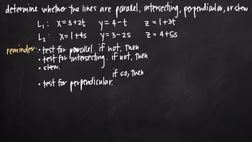PLAY PREVIEW

### Segments in this Video

#### Testing to Determine the Line Type (02:45)

FREE PREVIEW

To test for parallel lines, we use the equation for slope. If the slopes of the two lines are the same, then the lines are parallel. If the slopes of the two lines are not the same, then the lines are not parallel. Then we can test for intersecting, and then skew.

#### Testing for Parallel Lines(01:53)

To test for parallel, take the coefficient values on our parameters, which are also called our direction numbers.

#### Testing for Intersecting Lines(03:12)

We can test for intersecting lines by solving a system of simultaneous equations.

#### Skew Lines(02:42)

We've ruled out that they're not parallel or intersecting lines, so we know that they must be skewed.

#### Credits(00:00)

Credits

For additional digital leasing and purchase options contact a media consultant at 800-257-5126
(press option 3) or sales@films.com.

# Parallel, intersecting, skew and perpendicular lines

Part of the Series : Integral Calc: Calculus 3
 3-Year Streaming Price: \$49.95

Share

### Description

This video tutorial works through math problems/equations that address topics in Calculus 3, Partial Derivatives. This specific tutorial addresses Parallel, intersecting, skew and perpendicular lines.

Length: 11 minutes

Item#: BVL275735Closed Captioned